Algebraic Geometry Commons™

223 Full-Text Articles 268 Authors 56,862 Downloads69 Institutions

All Articles in Algebraic Geometry

223 full-text articles. Page 1 of 9.

Algebraic Methods For Proving Geometric Theorems, 2019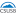California State University, San Bernardino

Algebraic Methods For Proving Geometric Theorems, Lynn Redman

Electronic Theses, Projects, and Dissertations

Algebraic geometry is the study of systems of polynomial equations in one or more variables. Thinking of polynomials as functions reveals a close connection between affine varieties, which are geometric structures, and ideals, which are algebraic objects. An affine variety is a collection of tuples that represents the solutions to a system of equations. An ideal is a special subset of a ring and is what provides the tools to prove geometric theorems algebraically. In this thesis, we establish that a variety depends on the ideal generated by its defining equations. The ability to change the basis of an ideal ...

Essential Dimension Of Parabolic Bundles, 2019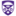The University of Western Ontario

Essential Dimension Of Parabolic Bundles, Dinesh Valluri

Electronic Thesis and Dissertation Repository

Essential dimension of a geometric object is roughly the number of algebraically independent parameters needed to define the object. In this thesis we give upper bounds for the essential dimension of parabolic bundles over a non-singular curve X of genus g greater than or equal to 2 using Borne's correspondence between parabolic bundles on a curve and vector bundles on a root stack. This is a generalization of the work of Biswas, Dhillon and Hoffmann on the essential dimension of vector bundles, by following their method for curves and adapting it to root stacks. In this process, we invoke ...

2019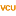Iowa State University

Topology And Dynamics Of Gene Regulatory Networks: A Meta-Analysis, Claus Kadelka

Biology and Medicine Through Mathematics Conference

No abstract provided.

Unifications Of Pythagorean Triple Schema, 2019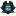East Tennessee State University

Unifications Of Pythagorean Triple Schema, Emily Hammes

Euclid’s Method of finding Pythagorean triples is a commonly accepted and applied technique. This study focuses on a myriad of other methods behind finding such Pythagorean triples. Specifically, we discover whether or not other ways of finding triples are special cases of Euclid’s Method.

2019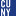The Graduate Center, City University of New York

On The Complexity Of Computing Galois Groups Of Differential Equations, Mengxiao Sun

All Dissertations, Theses, and Capstone Projects

The differential Galois group is an analogue for a linear differential equation of the classical Galois group for a polynomial equation. An important application of the differential Galois group is that a linear differential equation can be solved by integrals, exponentials and algebraic functions if and only if the connected component of its differential Galois group is solvable. Computing the differential Galois groups would help us determine the existence of the solutions expressed in terms of elementary functions (integrals, exponentials and algebraic functions) and understand the algebraic relations among the solutions.

Hrushovski first proposed an algorithm for computing the differential ...

2019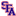Stephen F Austin State University

Data Parsing For Optimized Molecular Geometry Calculations, Luke Rens

The purpose of this project is to optimize and streamline to process of using ADF and ReaxFF. There is no efficient way to effectively add constraints to a compound and run it through ADF, take the ADF output and create a file that can be run through Reaxff, then take that Reaxff output and come to conclusions on it. To streamline this process, scripts were developed using Python to parse information out of data generated by ADF.

Enhanced Koszulity In Galois Cohomology, 2019The University of Western Ontario

Enhanced Koszulity In Galois Cohomology, Marina Palaisti

Electronic Thesis and Dissertation Repository

Despite their central role in Galois theory, absolute Galois groups remain rather mysterious; and one of the main problems of modern Galois theory is to characterize which profinite groups are realizable as absolute Galois groups over a prescribed field. Obtaining detailed knowledge of Galois cohomology is an important step to answering this problem. In our work we study various forms of enhanced Koszulity for quadratic algebras. Each has its own importance, but the common ground is that they all imply Koszulity. Applying this to Galois cohomology, we prove that, in all known cases of finitely generated pro-$p$-groups, Galois ...

Dense Geometry Of Music And Visual Arts: Vanishing Points, Continuous Tonnetz, And Theremin Performance, 2019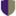Independent researcher, Palermo, Italy

Dense Geometry Of Music And Visual Arts: Vanishing Points, Continuous Tonnetz, And Theremin Performance, Maria Mannone, Irene Iaccarino, Rosanna Iembo

The STEAM Journal

The dualism between continuous and discrete is relevant in music theory as well as in performance practice of musical instruments. Geometry has been used since longtime to represent relationships between notes and chords in tonal system. Moreover, in the field of mathematics itself, it has been shown that the continuity of real numbers can arise from geometrical observations and reasoning. Here, we consider a geometrical approach to generalize representations used in music theory introducing continuous pitch. Such a theoretical framework can be applied to instrument playing where continuous pitch can be naturally performed. Geometry and visual representations of concepts of ...

Taking A Canon To The Adjunction Formula, 2019Georgia Southern University

Taking A Canon To The Adjunction Formula, Paul M. Harrelson

Electronic Theses and Dissertations

In this paper, we show how the canonical divisor of a graph is related to the canonical divisor of its subgraph. The use of chip firing and the adjunction formula for graphs ex- plains said relation and even completes it. We go on to show the difference between the formula for full subgraphs and that of non-full subgraphs. Examples are used to simplify these results and to see the adjunction formula in action. Finally, we show that though the adjunction formula seems simple at first glance, it is somewhat complex and rather useful.

2018Selected Works

Umsl Faculty Expertise

Research Specializations: Algebraic Geometry; Data Mining; Languages (English and Romanian); Machine Learning; Mathematical Aspects of String Dualities; Mathematical Physics; and R

2018Utah State University

Calculating The Cohomology Of A Lie Algebra Using Maple And The Serre Hochschild Spectral Sequence, Jacob Kullberg

All Graduate Plan B and other Reports

Lie algebra cohomology is an important tool in many branches of mathematics. It is used in the Topology of homogeneous spaces, Deformation theory, and Extension theory. There exists extensive theory for calculating the cohomology of semi simple Lie algebras, but more tools are needed for calculating the cohomology of general Lie algebras. To calculate the cohomology of general Lie algebras, I used the symbolic software program called Maple. I wrote software to calculate the cohomology in several different ways. I wrote several programs to calculate the cohomology directly. This proved to be computationally expensive as the number of differential forms ...

2018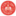Illinois State University

The Influence Of Canalization On The Robustness Of Finite Dynamical Systems, Claus Kadelka

Annual Symposium on Biomathematics and Ecology: Education and Research

No abstract provided.

Dual Perspectives On Desargues' Theorem, 2018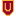Ursinus College

Dual Perspectives On Desargues' Theorem, Carl Lienert

Geometry

No abstract provided.

2018The Graduate Center, City University of New York

Galois Groups Of Differential Equations And Representing Algebraic Sets, Eli Amzallag

All Dissertations, Theses, and Capstone Projects

The algebraic framework for capturing properties of solution sets of differential equations was formally introduced by Ritt and Kolchin. As a parallel to the classical Galois groups of polynomial equations, they devised the notion of a differential Galois group for a linear differential equation. Just as solvability of a polynomial equation by radicals is linked to the equation’s Galois group, so too is the ability to express the solution to a linear differential equation in "closed form" linked to the equation’s differential Galois group. It is thus useful even outside of mathematics to be able to compute and ...

Webwork Problems For Linear Algebra, 2018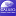University of North Georgia

Webwork Problems For Linear Algebra, Hashim Saber, Beata Hebda

Mathematics Ancillary Materials

This set of problems for Linear Algebra in the open-source WeBWorK mathematics platform was created under a Round Eleven Mini-Grant for Ancillary Materials Creation. The problems were created for an implementation of the CC-BY Lyrix open textbook A First Course in Linear Algebra. Also included as an additional file are the selected and modified Lyryx Class Notes for the textbook.

Topics covered include:

• Linear Independence
• Linear Transformations
• Matrix of a Transformation
• Isomorphisms
• Eigenvalues and Eigenvectors
• Diagonalization
• Orthogonality

Constructing Surfaces With (1/(K-2)^2)(1,K-3) Singularities, 2018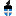Lawrence University

Constructing Surfaces With (1/(K-2)^2)(1,K-3) Singularities, Liam Patrick Keenan

Lawrence University Honors Projects

We develop a procedure to construct complex algebraic surfaces which are stable, minimal, and of general type, possessing a T-singularity of the form (1/(k-2)2)(1,k-3).

Mixed Categories Of Sheaves On Toric Varieties, 2018Louisiana State University and Agricultural and Mechanical College

Mixed Categories Of Sheaves On Toric Varieties, Sean Michael Taylor

LSU Doctoral Dissertations

In [BGS96], Beilinson, Ginzburg, and Soergel introduced the notion of mixed categories. This idea often underlies many interesting "Koszul dualities." In this paper, we produce a mixed derived category of constructible complexes (in the sense of [BGS96]) for any toric variety associated to a fan. Furthermore, we show that it comes equipped with a t-structure whose heart is a mixed version of the category of perverse sheaves. In chapters 2 and 3, we provide the necessary background. Chapter 2 concerns the categorical preliminaries, while chapter 3 gives the background geometry. This concerns both some basics of toric varieties as well ...

The Average Measure Of A K-Dimensional Simplex In An N-Cube, 2018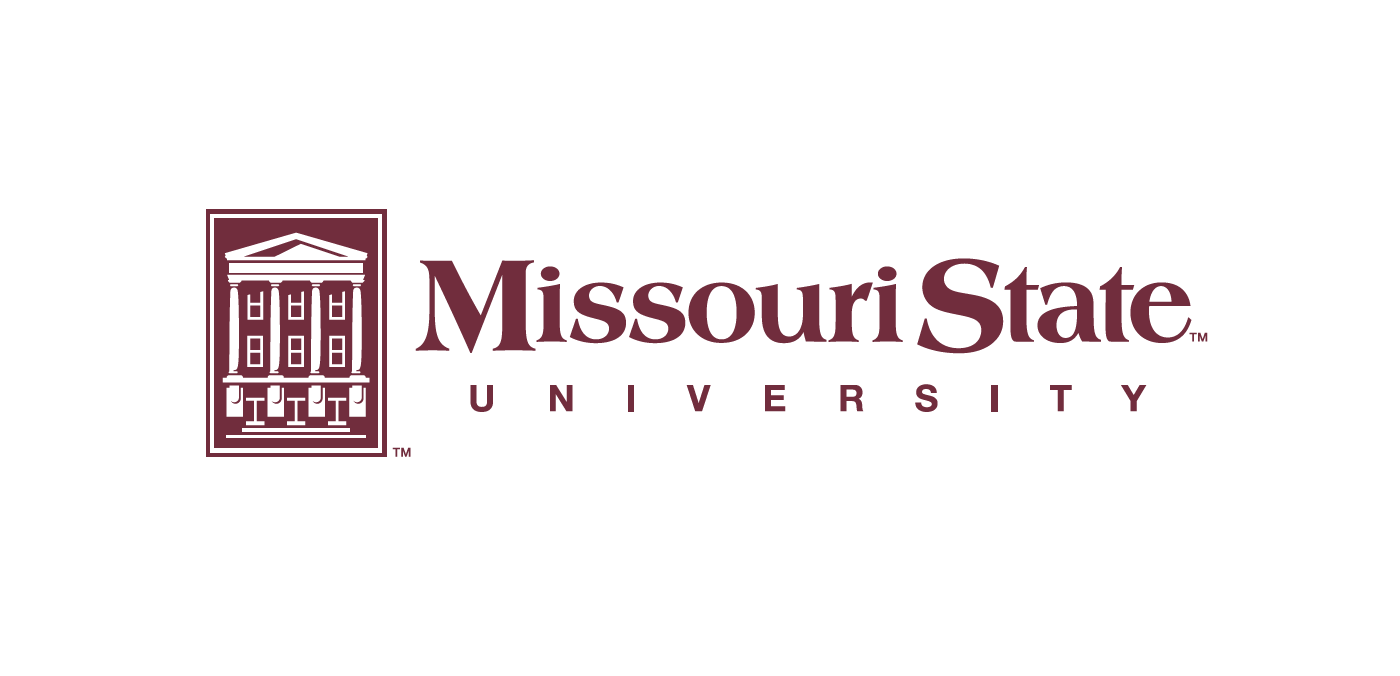Missouri State University

The Average Measure Of A K-Dimensional Simplex In An N-Cube, John A. Carter

Within an n-dimensional unit cube, a number of k-dimensional simplices can be formed whose vertices are the vertices of the n-cube. In this thesis, we analyze the average measure of a k-simplex in the n-cube. We develop exact equations for the average measure when k = 1, 2, and 3. Then we generate data for these cases and conjecture that their averages appear to approach nk/2 times some constant. Using the convergence of Bernstein polynomials and a k-simplex Bernstein generalization, we prove the conjecture is true for the 1-simplex and 2-simplex cases. We then develop a generalized formula for ...

Determinantal Representations Of Elliptic Curves Via Weierstrass Elliptic Functions, 2018Soochow University

Determinantal Representations Of Elliptic Curves Via Weierstrass Elliptic Functions, Mao-Ting Chien, Hiroshi Nakazato

Electronic Journal of Linear Algebra

Helton and Vinnikov proved that every hyperbolic ternary form admits a symmetric derminantal representation via Riemann theta functions. In the case the algebraic curve of the hyperbolic ternary form is elliptic, the determinantal representation of the ternary form is formulated by using Weierstrass $\wp$-functions in place of Riemann theta functions. An example of this approach is given.

Analytic Geometry And Calculus I, Ii, & Iii (Dalton), 2018Dalton State College

Analytic Geometry And Calculus I, Ii, & Iii (Dalton), Thomas Gonzalez, Michael Hilgemann, Jason Schmurr

Mathematics Grants Collections

This Grants Collection for Analytic Geometry and Calculus I, II, & III was created under a Round Six ALG Textbook Transformation Grant.

Affordable Learning Georgia Grants Collections are intended to provide faculty with the frameworks to quickly implement or revise the same materials as a Textbook Transformation Grants team, along with the aims and lessons learned from project teams during the implementation process.

Documents are in .pdf format, with a separate .docx (Word) version available for download. Each collection contains the following materials: